Previous: 7.2.3 Self Consistency Up: 7.2 The Open Von Next: 7.2.5 Existence But Non-Uniqueness

## 7.2.4 A Brute Force Approach: Parallelization with MPI

In a first step we reimplemented the methods described in [BAPGR02] and tried to apply them to the simulation of RTDs. Very soon it was realized, that the method lacks robustness. It is very difficult to achieve convergence. Only a few distinguished'' examples work. We were able to reach convergence for the device specified in [BAPGR02].

As a supposed cure to the robustness problem we implemented a full Newton method. Thereby we considered only the potential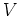as unknown, that is, we write the von Neumann-Poisson equation system in a form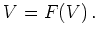(7.21)

Here the function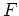consists in solving the set of Schrödinger equations to get the density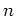as a function of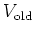and then solving the Poisson equation to derive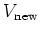as a function of. This eliminates the modes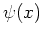from the system. The reason for the elimination is that without it we get a huge'' Jacobian matrix, as changing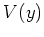on a single point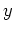has an influence on all valuesand the Jacobian becomes nonlocal.

Numerical costs for the calculation of the Jacobian ofare high. For each grid point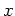we have to solve a set of Schrödinger equations. As there are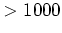grid points inand up to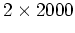points in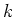-space, this can amount to solving up to four million Schrödinger equations for the calculation of the Jacobian. Though the implemented Schrödinger solver is highly optimized (Fortran code with a tridiagonal solver which was specialized for the application), this was too slow for practical purposes.

To overcome the cost constraints the calculation of the Jacobian was parallelized on a cluster of desktop PCs. Using MPI we followed a very restricted programming style to get a functional program, as debugging parallel applications is hard and mainly has to be done by extensive code inspection and occasionally by using good old printf statements. Unfortunately MPI itself lacks some fundamental functionalities. For instance, in MPI (at least in the standard version 1.x which we used), there are no routines available for checking if some node is up.

The implementation was kept modular. It can be combined with any nonlinear solver library. Most of these libraries allow the user to supply a function which calculates the Jacobian. We applied several well-known libraries to the von Neumann-Poisson problem, among them the Minpack and the Tensolve package. Furthermore we implemented and tried several textbook methods [Kel95]. We observed that non-Newton methods tended to violate the Dirichlet boundary conditions in the Poisson equation and high penalty weights had to be introduced in order to enforce fulfillment of the boundary conditions.

The full Newton solver behaved more robust than the simple'' approaches, which do not need the Jacobian. Especially, as code for the calculation of any expensive'' Jacobian matrix was available, we could check, that certain simple overrelaxation methods which are found in the literature can hardly work in practice for our examples, as the applied fixed-point iteration is not a contraction near the solution vector.

However, in the big picture we could not produce a robust implementation and in many cases we still cannot reach convergence. In cases where convergence is reached, the solution is numerically sound'', i.e., refinement of the grid or (reasonable) changes in the discretization give a result which is the same for all practical purposes.